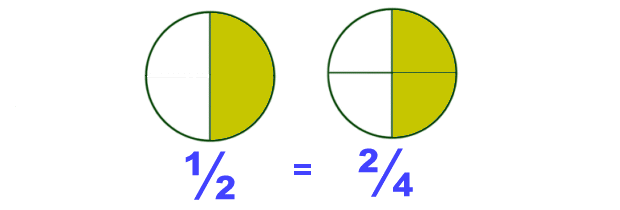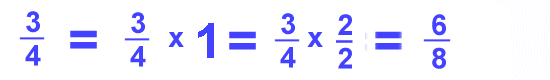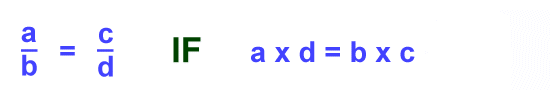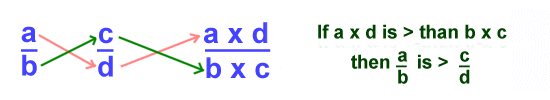Parents and Teachers: Support Ducksters by following us onor.

Kids Math

Equivalent Fractions

When fractions have different numbers in them, but have the same value, they are called equivalent fractions.

Let's take a look at a simple example of equivalent fractions: the fractions ½ and 2/4. These fractions have the same value, but use different numbers. You can see from the picture below that they both have the same value.How can you find equivalent fractions?

Equivalent fractions can be found by multiplying or dividing both the numerator and the denominator by the same number.

How does this work?

We know from multiplication and division that when you multiply or divide a number by 1 you get the same number. We also know that when you have the same numerator and denominator in a fraction, it always equals 1. For example:So as long as we multiply or divide both the top and the bottom of a fraction by the same number, it's just the same as multiplying or dividing by 1 and we won't change the value of the fraction.

Multiplication example:Since we multiplied the fraction by 1 or 2/2, the value doesn't change. The two fractions have the same value and are equivalent.

Division example:You can also divide the top and bottom by the same number to create an equivalent fraction as shown above.

Cross Multiply

There is a formula you can use to determine if two fractions are equivalent. It's called the cross multiply rule. The rule is shown below:This formula says that if the numerator of one fraction times the denominator of the other fraction equals the denominator of the first fraction times the numerator of the second fraction, then the fractions are equivalent. It's a bit confusing when written out, but you can see from the formula that it's fairly simple to work out the math.

If you get confused on what to do, just remember the name of the formula: "cross multiply". You are multiplying across the two fractions like the pink "X" shown in the example below.Comparing Fractions

How can you tell if one fraction is bigger than another?

In some cases it's pretty easy to tell. For example, after working with fractions for a while, you probably know that ½ is bigger than ¼. It's also easy to tell if the denominators are the same. Then the fraction with the larger numerator is bigger.

However, sometimes it's difficult to tell which is bigger just by looking at two fractions. In these cases you can use cross multiplication to compare the two fractions. Here is the basic formula:Here is an example:Key Things to Remember
• Equivalent fractions may look different, but they have the same value.
• You can multiply or divide to find an equivalent fraction.
• Adding or subtracting does not work for finding an equivalent fraction.
• If you multiply or divide by the top of the fraction, you must do the same to the bottom.
• Use cross multiplication to determine if two fractions are equivalent.

Back to Kids Math

Back to Kids StudyFollow us onor